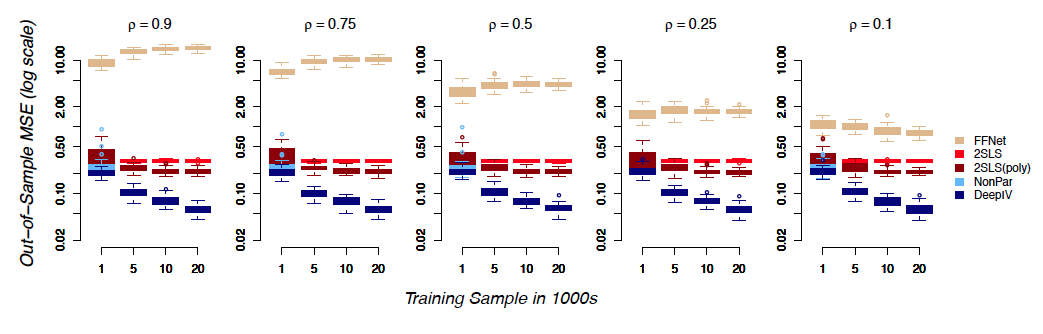# Econ-ML

A repository of papers that bring machine learning into economics and econometrics curated by John Coglianese, William Murdock, Ashesh Rambachan, Jonathan Roth, Elizabeth Santorella, and Jann Spiess

## Deep Learning for Instrumental Variables

Instrumental variables (IV) is one of the most important tools used to identify causal effects in economic research. If we can find a suitable instrument (relevant and plausibly exogenous), we can exploit it to identify the coefficient on the regressor of interest when we are worred about omitted variables bias. As presented in any standard econometrics textbook, the standard IV set-up makes strong, linearity assumptions. In particular, we assume that the endogenous regressor relates to the outcome of interest linearly and the instrument relates to the endogenous regressor linearly. For a variety of reasons, we may want to relax this functional form restriction. The literature on non-paramteric IV does so by replacing the simple linear regression set-up with flexible approximations using projections onto some set of basis functions or by using kernels. See Newey & Powell (2003) and Hall & Horowitz (2005) for examples. But, in the presence of high-dimensional data, kernel-based approaches may become computationally intractable and suffer from the curse of dimensionality. For this reason, Hartford et al. (2017) propose using deep neural networks as a flexible functional form in non-parametric IV. The authors estimate a treatment network, which is simply the first-stage in a two-stage IV procedure. It uses a deep neural network to parametrize the conditional distribution of the endogenous regressor given the instruments and other exogenous regressors. In the second stage, they construct an outcome network, which is another deep neural network used to approximate the conditional distribution of the outcome given the instrument and the other exogenous regressors weighted by the estimated relationship from the first stage. This is analagous to the second-stage of two-stage least squares, in which the outcome is regressed on the fitted values of the endogenous regressor from the first stage. Both networks are validated on held-out data at each stage of the estimation procedure.

Highlight: The authors compared the performance of Deep IV with 2SLS in a simulation. As the size of the training sample varied, the figure plots the mean square error of the predicted causal effect on held-out data relative to the true causal effect. As can be seen, for all sample sizes, Deep IV produces the most accurate causal estimates and its accuracy improves with the sample size.deep learning instrumental variables causal inference

Reviewed by Ashesh on .